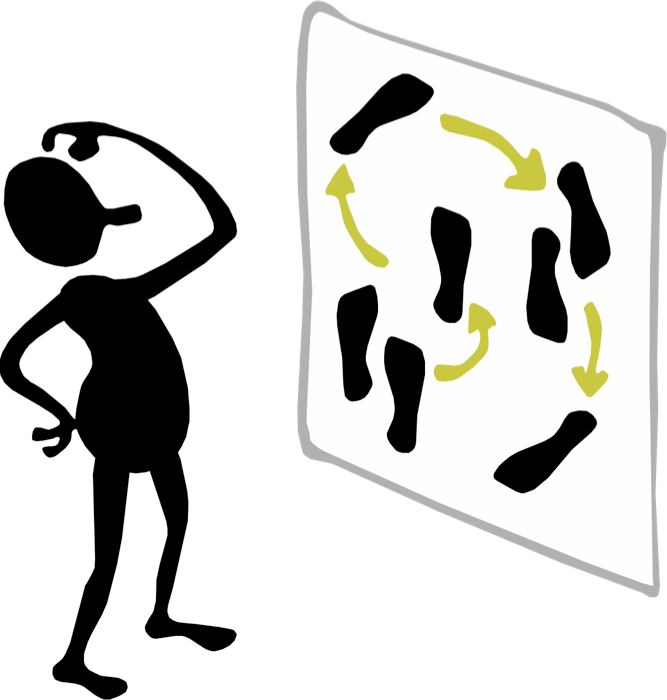## Clojure.Spec Workshop Part 2 - Data generationClojureRemote - February 2017
Yehonathan Sharvit
``````
(str (js/Date.))
``````

## Who am I?

• A mathematician
• A pragmatic theorist
• A coder
• A freak of interactivity
• Founded Audyx - an Audiology Startup with 30K LOCs in Clojurescript
• Author of KLIPSE
• A Clojure consultant## Agenda

• Data generation based on specs
• Specing functions
• Testing functions## KLIPSE - Code interactivity

Open this presentation in your computer for a collaborative live coding session: http://slides.klipse.tech/clojure-spec-cr17/part1.html#slide-3

(It works also on mobile)``````
(map inc [1 2 3])
``````

## Data Generation

For the upcoming parts, we need to require two additional namespaces

``````
(ns my.playground
(:require [clojure.test.check :as tc ]
[clojure.test.check.generators]
[clojure.test.check.properties :as prop :include-macros true]
[clojure.spec.alpha :as s]
[clojure.spec.test.alpha :as stest]
[clojure.spec.gen.alpha :as gen]))
``````

We can generate data samples out of our specs:

``````
(s/def ::big-integer (s/and integer?
#(> % 1000000)))
(s/def ::short-string (s/and string?
#(< (count %) 5)))
(s/def ::big-integer-or-short-string (s/or :int ::big-integer
:str ::short-string))
``````

Either with `gen/sample`:

``````
(gen/sample (s/gen ::big-integer) 5)
``````

Or with `s/exercise`:

``````
(s/exercise ::big-integer-or-short-string 5)
``````

## Data generation - maps

Why do we need to specify optional keys in a map?

``````
(gen/sample (s/gen (s/keys :req [::big-integer])) 5)
``````
``````
(gen/sample (s/gen (s/keys :req [::big-integer] :opt [::short-string])) 5)
``````

## Functions - Spec'ing the arguments

You can describe the shape of the args and return value of your functions

Let's imagine we have an addition function where the result is bounded:

``````
(defn bounded-addition [a b {:keys [upper lower]}]
(cond-> (+ a b)
lower (max lower)
upper (min upper)))
``````

Here are the initial specifications of our function:

``````
(s/def ::upper number?)
(s/def ::lower number?)
:args (s/cat :a number? :b number? :boundaries (s/keys :req-un [::upper ::lower])))
``````

What happens when one passes the wrong arguments?

``````
``````

You can `instrument` and `unstrument`:

``````
``````

What is the default - `instrument` or `unstrument`?

## Functions - exercise-fn

Let's check how our function behaves with valid random arguments

``````
``````

## Functions - Going further

We can also specify:

1. The returned value - a number
2. The relationships between different parts of the input - upper bound not smaller than lower bound
3. The relationships between the input and the returned value - returned value between lower and upper bounds

Let's imagine we have a multiplication function where the result is bounded:

``````
(defn bounded-multiplication [a b {:keys [upper lower]}]
(-> (* a b)
#_(max lower)
#_(min upper)))
``````

Here are the full specifications of our function:

``````
(s/def ::upper number?)
(s/def ::lower number?)
(s/fdef bounded-multiplication
:args (s/and (s/cat :a number? :b number? :boundaries (s/keys :req-un [::upper ::lower]))
#(>= (-> % :boundaries :upper) (-> % :boundaries :lower)))
:ret number?
:fn #(<= (-> % :args :boundaries :lower) (:ret %) (-> % :args :boundaries :upper)))
``````

``````
(-> (stest/check `bounded-multiplication {::tc/opts {:num-tests 10}})
first
stest/abbrev-result)
``````

## References## Questions?